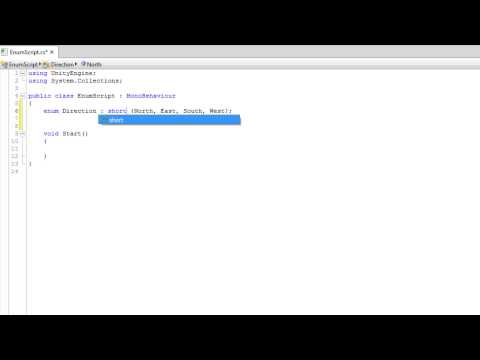# 列挙

#### 難易度: 初級## 列挙

### Code snippet

``````using UnityEngine;
using System.Collections;

public class EnumScript : MonoBehaviour
{
enum Direction {North, East, South, West};

void Start ()
{
Direction myDirection;

myDirection = Direction.North;
}

Direction ReverseDirection (Direction dir)
{
if(dir == Direction.North)
dir = Direction.South;
else if(dir == Direction.South)
dir = Direction.North;
else if(dir == Direction.East)
dir = Direction.West;
else if(dir == Direction.West)
dir = Direction.East;

return dir;
}
}``````
``````#pragma strict

enum Direction {North, East, South, West};

function Start ()
{
var myDirection : Direction;

myDirection = Direction.North;
}

function ReverseDirection (dir : Direction) : Direction
{
if(dir == Direction.North)
dir = Direction.South;
else if(dir == Direction.South)
dir = Direction.North;
else if(dir == Direction.East)
dir = Direction.West;
else if(dir == Direction.West)
dir = Direction.East;

return dir;
}``````
``````import UnityEngine
import System.Collections

public class EnumScript(MonoBehaviour):

private enum Direction:

North

East

South

West

private def Start():
myDirection as Direction

myDirection = Direction.North

private def ReverseDirection(dir as Direction) as Direction:
if dir == Direction.North:
dir = Direction.South
elif dir == Direction.South:
dir = Direction.North
elif dir == Direction.East:
dir = Direction.West
elif dir == Direction.West:
dir = Direction.East

return dir``````